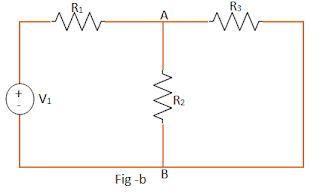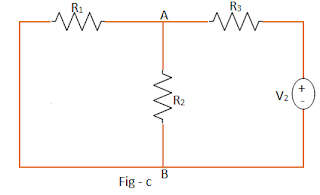# Explanation of Superposition Theorem

This theorem is applicable for linear and bilateral networks.  Let us see the statement of the theorem.

Statement : In an any multisource complex network contains  linear bilateral  elements, the voltage across or current pass through any given element of the network is identical to the algebraic sum of the individual voltages or currents, produced separately across or in that element by each source acting separately, when all the residual sources are replaced by  their relevant internal resistances.

This theorem is also called as Superposition principle. In other terms, it can be stated as; the response in any element of linear, bilateral network consisting more than one source is the sum of the responses produced by the sources, each acting independently. The response means the voltage across the element or the current in the element. The superposition theorem does not apply to the power as power is proportional to square of the current, which is not a linear function.

### Explanation of Superposition Theorem

Consider a network, shown in the Fig-a, having two voltage   sources V1 and V2.Let us calculate, the current in branch A-B of the network, using superposition theorem
Step 1) According to Superposition theorem, consider each source individually.  Let source V1 volts is acting independently. At this time, other sources should be replaced by internal impedances.
But as internal impedance of V2 is not given, the source V2 should be replaced by short circuit. Thus circuit becomes, as shown in the Fig-b.Using any of the network reduction techniques discussed earlier, obtain the current through branch A-B i.e. IAB due to source V1 alone.
Step 2) Now consider source V2 volts alone, with V1 replaced by a short circuit, to obtain the current through branch A-B. The equivalent circuit is shown in the Fig-c.Obtain IAB due to V2 alone, by using any of the network reduction techniques discussed
Step 3) According to the Superposition theorem, the sum current through the branch A-B is sum of the currents through branch A-B produced by every source acting separately.

#### Steps to Apply Superposition Theorem

Step 1: Decide on a single source acting alone. Short the other voltage sources and open the current sources, if internal resistances are not known. If known, replace them by their internal resistances.
Step 2: Find the current through or the voltage across the required element, due to the source under consideration, using a suitable network simplification technique.
Step 3: Repeat the above two steps for all the sources
Step 4: Add the individual effects produced by individual sources, to obtain the total current in or voltage across the element.0 CommentsComments